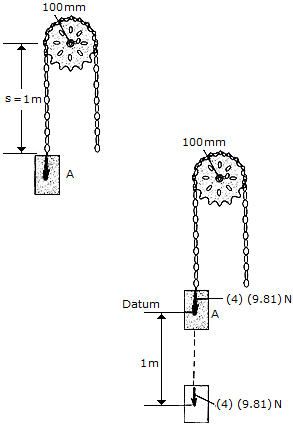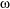# Engineering Mechanics - PKRB: Work and Energy - Discussion

### Discussion :: PKRB: Work and Energy - General Questions (Q.No.4)

4.A chain that has a negligible mass is draped over a sprocket which has a mass of 2 kg and a radius of gyration of kO = 50 mm. If the 4-kg block A is released from rest in the position shown, s = 1 m, determine the angular velocity which the chain imparts th the sprocket when s = 2 m.

 [A].= 44.3 rad/s [B].= 39.6 rad/s [C].= 41.8 rad/s [D].= 59.1 rad/s

Explanation:

No answer description available for this question.

 Paul said: (Feb 7, 2015) I am not sure if I am missing something with this question. I use the potential energy from the mass to determine the kinetic energy imparted on the sprocket. I use 4kg for the potential energy and then use 2 kg for the kinetic energy imparted on the sprocket. From there I get a velocity and simply divide by the radius of 0.05 m to find the angular velocity but I don't get answer C.

 Gege said: (Jun 9, 2017) T2= 1/2mv^2+1/2Iw^2, = 1/2(4)(wr)^2+1/2(0.01)w^2, = 0.01w^2. T1+V1= T2+V2. 0+ 19.62N = 0.01w^2 + 0. w = 44.29 rad/s.

 Ivan said: (Dec 4, 2017) T2= 1/2mv^2+1/2Iw^2, = 1/2(4)(w*0.1)^2+1/2(0.005)w^2, = 0.0225w^2. T1+V1=T2+V2. 0+0=0.0225w^2-39.24N. w=41.76 rad/s.

 Mohd Farihsan Bin Zamzuri said: (May 12, 2020) If the angular velocity is too high at the instant s= 2m, revise the set up to reduce the angular velocity without changing the mass of block A.